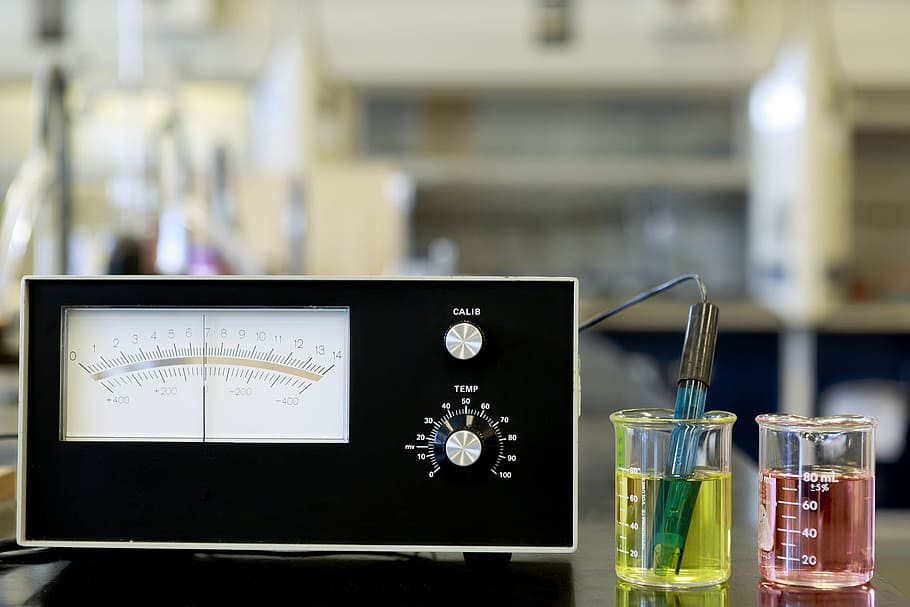# What is the Difference Between Acidimetry and Alkalimetry

The main difference between acidimetry and alkalimetry is that acidimetry is the process of measuring the concentration of an acid in a given sample, whereas alkalimetry is the process of determining the concentration of a base in a sample.

Acidimetry and alkalimetry are two analytical techniques in chemistry to determine the concentration of acids and bases.

### Key Areas Covered

1. What is Acidimetry
– Definition, Function, Titrants
2. What is Alkalimetry
– Definition, Function, Titrants
3. Similarities – Acidimetry and Alkalimetry
– Outline of Common Features
4. Difference Between Acidimetry and Alkalimetry
– Comparison of Key Differences

### Key Terms

Acidimetry, Alkalimetry## What is Acidimetry

In the field of analytical chemistry, acidimetry is a fundamental technique used to determine the concentration of acids in a given sample. By employing a titration method, acidimetry allows for precise and accurate measurements of acid concentration. Acidimetry relies on the principle of neutralization, where a standard solution of a base known as a titrant is added to the acid solution. The titrant reacts with the acid until the equivalence point is reached, indicating that the acid has been completely neutralized.

The equivalence point is typically determined using an indicator or a pH meter. In addition, the volume of titrant required to reach the equivalence point is used to calculate the concentration of the acid.In acidimetry, strong bases are commonly used as titrants due to their ability to completely neutralize acids. Sodium hydroxide and potassium hydroxide are popular choices for acidimetric titrations. These bases have known concentrations and are carefully standardized to ensure accuracy and reliability in acid concentration determination. Moreover, the selection of the titrant depends on the nature of the acid being analyzed.

Indicators play a main role in acidimetry by providing a visual signal to indicate the endpoint of the titration. Acid-base indicators undergo a color change within a specific pH range, which corresponds to the neutralization of the acid. Furthermore, commonly used indicators in acidimetry include phenolphthalein, methyl orange, bromothymol blue, and methyl red. The choice of an indicator depends on the expected pH range of the acid being titrated.

Furthermore, acidimetry has extensive applications in various fields, including quality control, environmental analysis, education and research, and acidimetric assays.

## What is Alkalimetry

Alkalimetry is an analytical method used to determine the concentration of bases in a solution. It involves titrating a known volume of the base solution with a standardized acid until reaching the endpoint. Generally, an indicator or pH meter detects the endpoint.

In alkalimetry, a strong acid such as hydrochloric acid or sulfuric acid is added gradually to the base solution. As the acid reacts with the base, the pH of the solution decreases. The titration continues until the pH reaches a specific value, usually indicated by a color change of the indicator or a pH meter reading.The concentration of the base in the solution is calculated based on the volume of the acid titrant required to reach the endpoint. The stoichiometry of the reaction between the acid and the base is also considered during the calculation. In fact, knowing the concentration of the acid titrant and the volume used can determine the base’s concentration.

Furthermore, alkalimetry has applications in various fields, including pharmaceutical, environmental, and industrial applications, to measure the concentration of bases in different samples. In brief, it is a precise and reliable method and allows for  the accurate determination of base concentrations in solutions

## Similarities Between Acidimetry and Alkalimetry

• Acidimetry and alkalimetry rely on the principle of neutralization.
• Both acidimetry and alkalimetry are quantitative analytical methods.
• Both involve neutralization reactions between the acid and the base.

## Difference Between Acidimetry and Alkalimetry

### Definition

Acidimetry is the process of measuring the concentration of an acid in a given sample, whereas alkalimetry is the process of determining the concentration of a base in a sample.

### Substances Analyzed

Acidimetry is the analytical method used to determine the concentration of acids in a solution, while alkalimetry is used to determine the concentration of bases in a solution.

### Titrants

Acidimetry uses a strong base as the titrant, whereas alkalimetry uses a strong acid as the titrant.

### Conclusion

The main difference between acidimetry and alkalimetry is that acidimetry is the process of measuring the concentration of an acid in a given sample, whereas alkalimetry is the process of determining the concentration of a base in a sample.

##### Reference:

1. “Definition, Form, and Examples of Alkalimetric Titration.” Hyprowira.
2. Lipsa, Samal. “ACIDIMETRY AND ALKALIMETRY TITRATION” Centurion University.

##### Image Courtesy

1. “Test Tube Lab” (CC0) via Pixabay
2. “Analog pH meter with electrode, pH 7 and 4 buffers., test, acid, HD wallpaper” (CC0) via Wall Paper Flare.